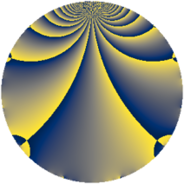# Properties

 Label 930.2.rLevel $930$ Weight $2$ Character orbit 930.r Rep. character $\chi_{930}(119,\cdot)$ Character field $\Q(\zeta_{6})$ Dimension $128$ Newform subspaces $2$ Sturm bound $384$ Trace bound $2$

# Related objects

## Defining parameters

 Level: $$N$$ $$=$$ $$930 = 2 \cdot 3 \cdot 5 \cdot 31$$ Weight: $$k$$ $$=$$ $$2$$ Character orbit: $$[\chi]$$ $$=$$ 930.r (of order $$6$$ and degree $$2$$) Character conductor: $$\operatorname{cond}(\chi)$$ $$=$$ $$465$$ Character field: $$\Q(\zeta_{6})$$ Newform subspaces: $$2$$ Sturm bound: $$384$$ Trace bound: $$2$$

## Dimensions

The following table gives the dimensions of various subspaces of $$M_{2}(930, [\chi])$$.

Total New Old
Modular forms 400 128 272
Cusp forms 368 128 240
Eisenstein series 32 0 32

## Trace form

 $$128q + 128q^{4} - 8q^{9} + O(q^{10})$$ $$128q + 128q^{4} - 8q^{9} + 4q^{10} + 128q^{16} + 8q^{19} + 4q^{25} + 36q^{31} + 12q^{34} - 8q^{36} - 16q^{39} + 4q^{40} + 14q^{45} + 60q^{49} - 16q^{51} + 128q^{64} - 16q^{66} - 20q^{69} + 32q^{70} - 48q^{75} + 8q^{76} - 60q^{79} - 24q^{81} + 4q^{90} - 8q^{94} - 204q^{99} + O(q^{100})$$

## Decomposition of $$S_{2}^{\mathrm{new}}(930, [\chi])$$ into newform subspaces

Label Dim. $$A$$ Field CM Traces $q$-expansion
$$a_2$$ $$a_3$$ $$a_5$$ $$a_7$$
930.2.r.a $$64$$ $$7.426$$ None $$-64$$ $$0$$ $$-2$$ $$0$$
930.2.r.b $$64$$ $$7.426$$ None $$64$$ $$0$$ $$2$$ $$0$$

## Decomposition of $$S_{2}^{\mathrm{old}}(930, [\chi])$$ into lower level spaces

$$S_{2}^{\mathrm{old}}(930, [\chi]) \cong$$ $$S_{2}^{\mathrm{new}}(465, [\chi])$$$$^{\oplus 2}$$